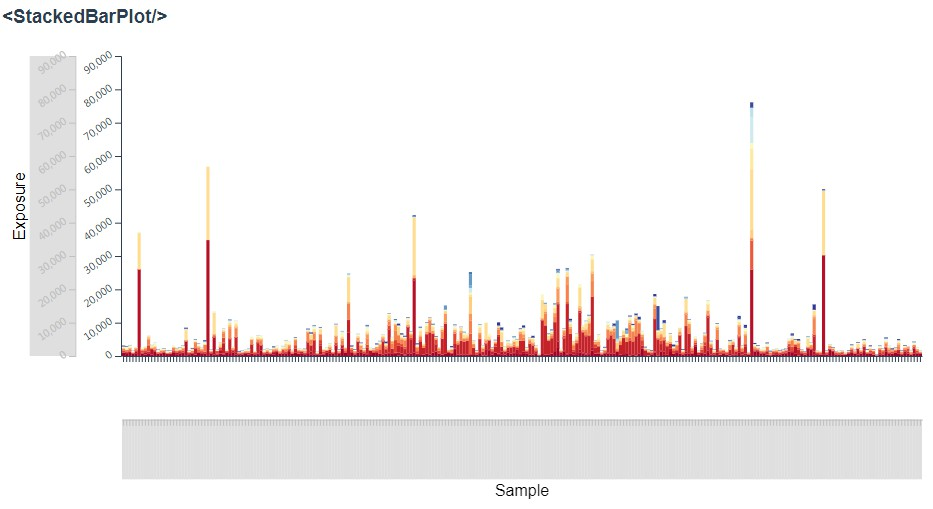/ Charts

# Declarative and interactive Vue plot components built with D3## vue-declarative-plots

This repository will serve as a place for reusable Vue plot components (built with D3). These components were developed for use in iMuSE.

Coming soon

### Development

Install dependencies:

``````yarn
``````

Serve for development at http://localhost:8080:

``````yarn run serve
``````

Build for production (generates `/dist` and `/examples`):

``````yarn run build
``````

Run tests with jest:

``````yarn run test
``````

Compile documentation with documentationjs:

``````yarn run docs
``````

### Plot Components

• BarPlot :white_check_mark:
• StackedBarPlot :white_check_mark:
• ScatterPlot :white_check_mark:
• GenomeScatterPlot :white_check_mark:
• GenomeStackedBarPlot
• GenomeTrackPlot
• TrackPlot :white_check_mark:
• MultiTrackPlot
• BoxPlot :white_check_mark:
• MultiBoxPlot :white_check_mark:
• ViolinPlot

### Axis Components

• Axis :white_check_mark:
• GenomeAxis :white_check_mark:
• DendrogramAxis

### Other Components

• PlotContainer :white_check_mark:
• Legend
• SortOptions :white_check_mark:

### Goals:

• [x] props should declare visual encodings of data
• example: the StackedBarPlot component will accept the following props:
``````x="sample_id"
y="exposure"
c="signature" // color
``````
• [x] props should declare where to find the data
• data will never be passed directly to a plot - instead it will be globally accessible by a key
• for now, assume data is stored in a global JSON object with `<key>: <data>` mappings
• example: for a dataset with key `exposures_data`, the following prop would specify to a plot component that this dataset should be used:
``````data="exposures_data"
``````
• [x] props should declare where to find the scales
• [x] scales will be ES6 classes with different APIs depending on whether categorical, continuous, etc...
• [x] scales will always expose a domain
• [x] scales will always expose a domain-var-to-color function
• [ ] the color scale (or even individual colors) should also be able to be changed programmatically
• [x] scales will always expose a domain-var-to-human-readable-text function
• [x] categorical scales will always expose a sort function that takes in a specification of the data that will define the ordering
• [x] scales will always expose a filter function (and a corresponding filter-reset function)
• if categorical, this will accept an array of new values
• if continuous, this will accept a `[min, max]` array
• if binary, this will accept a boolean value
• scales should contain all of the information necessary to draw a legend
• scales will never be passed directly to a plot - instead they will be globally accessible by a key
• for now, assume scales are stored in a global JSON object with `<variable>: <scale>` mappings
• example: for a variable `sample_id`, the following prop would specify to a plot component that this scale object should be used for the x axis:
``````x="sample_id"
``````
• [x] plots should assume which type of scale is on which axis
• for example, a bar plot (with vertical bars) would assume a continuous y scale and a categorical x
• [x] events should alert plots when a scale has been mutated
• these alerts should specify which scale has been updated using a key
• plot components should listen for these updates and re-draw if necessary
• scales may be mutated upon filter or zoom
• [x] data should be immutable
• even small variations of data sets should be stored in a separate `DataContainer` instance
• [x] plots should NOT draw their own axes
• axes should be independent of plots
• axes should be contained in their own components
• [x] axes should accept props specifying which scale to use, and where to draw
• example:
``````variable="sample_id"
side="bottom"
``````
• [x] axes should be brush-able
• but also should have the option to disable brushing
• brushing along an axis should trigger a zoom event
• axes, if their corresponding scale has been "zoomed", should display a "zoomed out"/full view beside the "zoomed-in" view in order to show context
• categorical axes should snap
• [x] plots and axes should accept `width` and `height` props
• container components should be responsible for keeping plot and axis props in sync if they are dynamic
• example:
``````:pWidth="windowWidth"
:pHeight="300"
``````
• [x] plots should accept margin props
• container components should be responsible for keeping margin props in sync if they are dynamic
• example:
``````:pMarginTop="10"
:pMarginLeft="50"
:pMarginRight="20"
:pMarginBottom="0"
``````
• [x] plots should emit click events, specifying variables in a predefined order to a prop-supplied callback
• example:
``````:clickHandler="chooseSample" // will be called with chooseSample(x, y, c)
``````
• [x] plots should have tooltips
• tooltips should obtain human-readable variable names from the appropriate scale
• [x] plots should dispatch applicable hover events
• dispatching should be done through the scale
• example:
• hovering on a section of a bar on a stacked bar plot would cause dispatches for (at least) the `x` and `color` variables
• [x] the internals of the drawing of the plots should be abstracted away as much as possible
• details of SVG, canvas, etc. implementation should be contained
• [x] all meaningful interactions will be stored in a history stack
• meaningful interactions: scale filter/zoom/sort
• will allow backward(undo)/forward(redo) operations
• will allow "replaying" of the user's interactions
• will allow sharing of a user session, so that others may replay or step through the interactions
• but this should also be optional, for example if the user chooses not to supply the stack to a plot via prop